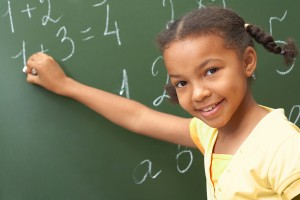# Subtract vs. Minus–The Language of MathHere's an equation:  8-2=6

Conventionally we would say, "Eight minus two equals six."

Is it incorrect to say "Eight subtract two equals six?"
My son said the latter at school, as I have done while working with him, and was told that it is incorrect by his teacher.
Is it?  Is it also wrong to say "Eight add two equals ten"?  Are the words minus/subtract or add/plus interchangeable when we read equations or is it wrong to say anything but plus or minus?
I posed that question to a math discussion group and got a range of responses.  Some people said that it's grammatically incorrect to say subtract because it's a verb and minus is not and there is already one verb, equals, in the sentence.
Others said that either is correct but subtract is more precise and that mathematical expressions don't have to conform to english grammatical structure.  It was quite a lively debate.
The clearest most concise response I received came from Alison Coates of the Middle School Mathematics Institute.  Here's what she had to say:

Perhaps this is a quibble, but math is not easily discussed in lay language properly.   That is the reason we use symbols for math: the symbols of math are the "language" of math, and our collective unwillingness in the US to prepare students for symbolic manipulation is a big reason that they have more and more errors, until their errors in reasoning swamp truth.

Without any verbalization, 8 - 2 = 6 is precise, succinct, and correct. It is distinct from 2 - 8 = -6.

It is only when we try to verbalize this that we get into trouble.

There are a couple related issues here worth teasing out:

1. We should teach children to "read" the above equation as a transliteration of the symbols, so someone on hearing the verbalization of the equation writes down the correct one.

In English, we verbalize the above as "eight minus 2 equals 6".  While we may wish to discuss or explain sums, differences, etc., in the end, a student must know how to name these symbols properly so they can write down the appropriate expression correctly without confusion.  That symbol is a minus (sign), and + is a plus (sign). We read 3 x 4 = 12 as "three times four equals 12."  This is separate from how we converse about these concepts.

2.  Precision matters.  The problem with various English expressions and their inexactness as a substitute for mathematical precision is often only seen over time, far too late to fix misconceptions.

I have met many 7th graders who do not know decimals are fractions.  They have never been told "a decimal is a fraction with a power of ten as the denominator".  They almost always, to a one, verbalize the symbol 4.56 as "four point five six".  They have no idea that .56 is the fraction fifty-six hundredths.  Being forced to say it properly until absolute demonstration of mastery of place value in decimals and of recognition of decimal as fraction is critical.

Likewise, I have met many second graders who can not solve, “Anne has two more cars than her brother Charlie.  Anne has 8.  How many does Charlie have?” because they have been taught that subtract is "take away".  But there is nothing here being taken away in their view.

I'm sure most members here have thoroughly hashed out the errors in "a fraction is a part of a whole"--and you may think this is a different kind of language error, but to most teachers, it isn't.  If you as a teacher are afraid to introduce the definitions of equality or commutative properties, you aren't going to be comfortable with a fraction defined on a number line precisely.  (For those who don't know what's wrong with "a fraction is part of a whole", incredibly short answer: it leads student to think that all fractions are less than 1.  Because how can more than a whole be part of a whole?   What's 9/8--what's the part, what's the whole?   Most students will answer "it's really 1 1/8", and they think only 1/8 is the fraction.)

My "favorite" example of how misuse of language completely ruins a child's understanding of math is of a 6 yr old girl in a (nominally?) Montessori program who was bringing home page after page of 3 digit by 3 digit vertical algorithm addition problems, and doing them correctly.  But then her mother asked her to solve (horizontally) 9 + 2 = ?

and she said (yes, direct quote): "Eleven, but the answer can't be greater than 9."

She could explain place value exchanges at "the bead store", and she could compute with carrying correctly, but when the teacher taught her about adding, the teacher failed to distinguish between *we only have 10 digits to write with" and "the total number of allowed objects can never be greater than nine".

So the girl went about adding e.g.

372

+159

correctly

and simultaneously thought 9 + 2 = 11 was illegal.

Getting these details right for a child is difficult work, but they matter very much.

Allison Coates

Middle School Mathematics Institute

www.msmi-mn.org